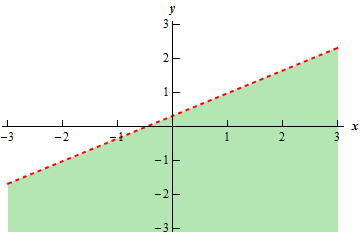Paul's Online Notes
Home / Calculus III / 3-Dimensional Space / Functions of Several Variables
Show Mobile Notice Show All Notes Hide All Notes
Mobile Notice
You appear to be on a device with a "narrow" screen width (i.e. you are probably on a mobile phone). Due to the nature of the mathematics on this site it is best views in landscape mode. If your device is not in landscape mode many of the equations will run off the side of your device (should be able to scroll to see them) and some of the menu items will be cut off due to the narrow screen width.

### Section 12.5 : Functions of Several Variables

2. Find the domain of the following function.

$f\left( {x,y} \right) = \ln \left( {2x - 3y + 1} \right)$ Show Solution

There really isn’t all that much to this problem. We know that we can’t have negative numbers or zero in a logarithm so we’ll need to require that whatever $$\left( {x,y} \right)$$ is it will need to satisfy,

$2x - 3y + 1 > 0$

Since this is the only condition we need to meet this is also the domain of the function.

Let’s do a little rewriting on this so we can attempt to sketch the domain.

$2x + 1 > 3y\hspace{0.5in}\Rightarrow \hspace{0.5in}y < \frac{2}{3}x + \frac{1}{3}$

So, it looks like we need to be below the line above. The domain is illustrated by the green area in the sketch below.Note that we dashed the graph of the “bounding” line to illustrate that we don’t take points from the line itself.